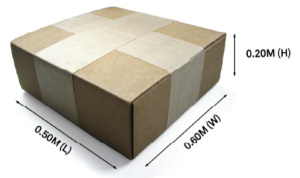# Calculate the Weight

Calculating the chargeable weight for your shipment will be based on two factors:

1. The actual (dead) weight of the shipment; or
2. The volumetric (cubic) weight of the shipment

Whichever figure is the greater; actual weight or volumetric weight will be used to calculate your freight charges.

Standard volumetric rate is 1 cubic metre = 250 kilograms

The reason that the chargeable weight is calculated this way is because typically large items with a light overall weight will take up more space on an aircraft than a small, heavy item.

The following is an example of a basic calculation:

###Calculate the following two factors:

 Shipment (dead) weight: 30.0 kg Volumetric (cubic) weight: L X W X H in metres 0.50 X 0.60 X 0.20 = 0.060 m3

### Step 2

Convert the volumetric (cubic) weight to the equivalent weight
(based on 1m3 = 250kg)    0.060 m3 X 250 kg = 15.0kg